# Civil Engineering - UPSC Civil Service Exam Questions

36.

Following data refer to precise measurement of an angle A on the field :
(i) 2A = 20°10' - weight 2
(ii) 4A = 40°10' - weight 3
What is the most probable value of angle A ?

 A. 10°01'06" B. 10°02'07" C. 10°02'51" D. 10°22'51"

Explanation:

No answer description available for this question. Let us discuss.

37.

A torsion member is fabricated from two concentric thin tubes. At the ends, the tubes are welded to rigid discs so that both the tubes are twisted as a unit. The radius of the outer tube is 2r and that of the inner tube is r. If the shear stresses developed in the outer tube is τ, then the shear stress in the inner tube will be.

 A. τ B. 0.25 τ C. 0.75 τ D. 0.5 τ

Explanation:

No answer description available for this question. Let us discuss.

38.

Figure given below shows a fixed beam of steel: At the point of collapse, the value of load W will be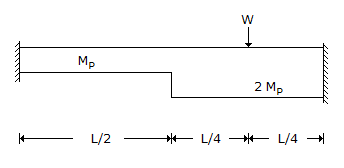A.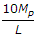B.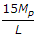C.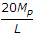D.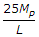Explanation:

No answer description available for this question. Let us discuss.

39.

A mild steel flat of width 120 mm and thickness 10 mm is bent into an arc of a circle of radius 10 m by applying a pure moment 'M' If E is 2 x 105 N/mm2, then the magnitude of the pure moment 'M' will be

 A. 2 x 106 N-mm B. 2 x 105 N-mm C. 0.2 x 105 N-mm D. 0.2 x 104 N-mm

Explanation:

No answer description available for this question. Let us discuss.

40.

The deflection at the free end of a uniformly loaded cantilever of length 1 m is 7.5 mm. What is the slope at the free end ?